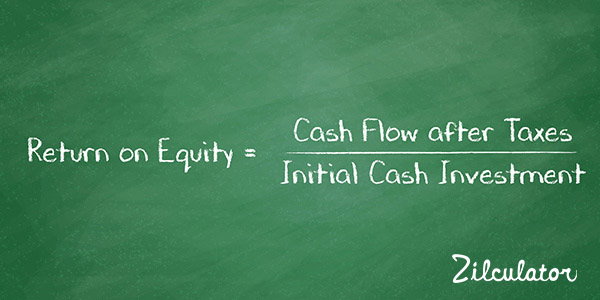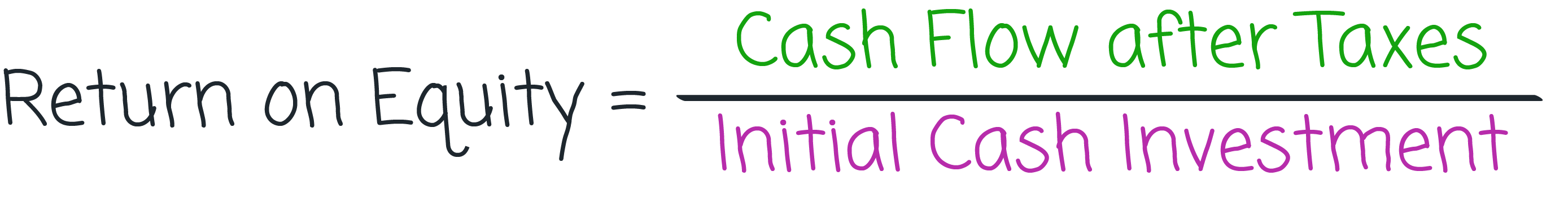Return on Equity in Real Estate

Formula & DefinitionThere are two ways of approaching the topic of return on equity (ROE) as it applies to real estate investments. In each of them, "return" has the same meaning: cash flow after taxes (CFAT). What differs is the meaning of "equity". In the traditional method, the equity is your initial cash investment, this is then very similar to Cash-on-Cash return calculation, with the difference of Cash Flow before Taxes in the Cash-on-Cash return. In the alternative technique, it is you initial cash plus the additional equity that has built up due to ammortization of the mortgage and to increase in the value of the property.

The ROE is expressed as a percentage and typically is calculated for the first year only, however it can tell some interesting information in the following years as well.

Zilculator helps real estate professionals calculate return on equity easily. Never use a spreadsheet again! Analyze your own property or create investment reports for your clients.

• Sales and Rental comps

How to Calculate Return on Equity in Real Estate

1. Determine cash flow after taxes for the given year (usually Year 1).
2. Divide the first number by initial cash investment using the formula below: